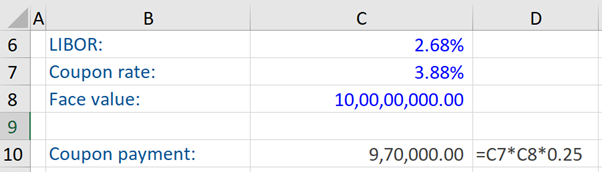## What are “Coupon Types”?

Bonds are a form of debt instrument, which are a contractual agreement between the issuer of the bond and the bondholders. Bonds can have fixed or floating rate coupon bonds. These bonds periodically pay a nominal rate of interest (coupons) –  typically annually or semiannually during the lifetime of the bond. In fixed-rate bonds, the coupon rate does not change during the life of the bond, whereas, in the case of floating rate bonds, the coupon rate is reset at certain times or periodically (for example, semi-annually) and the next coupon can be higher or lower than the previous one.

## Key Learning Points

• There are several bond coupon types – fixed and floating rate bonds, and there is a special type of fixed-rate bond – a zero-coupon bond
• Unlike a fixed rate bond, in a floating rate bond, the next coupon can be higher or lower than the previous coupon

## Coupon Types – Fixed Rate and Floating Rate Bonds

Between the bond issuance date and maturity date, bond issuers make regular interest payments to the bond investors. The vast majority of bonds are fixed-rate bonds. This means that coupons are set at issuance and do not change over the life of the bond.

With a fixed-rate bond such as a 10-year, 3% coupon annual bond, the investor knows exactly what interest payments will be received every year until maturity. This is assuming that the bond issuer does not default at any point in time.

There is a special type of fixed-rate bond called a zero-coupon bond. In this case, there is no interest payment between the issuance of the bond and maturity. So, they “pay” a fixed coupon of 0%. This does not mean that there is no return for bondholders, as zero-coupon bonds are usually sold at a discounted price but repaid at 100% at maturity. So, it is this difference between the purchase and redemption price that investors will realize over the life of the bond.

For example, we have a 10-year zero-coupon bond issued at a price of 74.51% and no interest will be paid over the 10-year term, and the bond will be repaid or redeemed at 100%. Therefore, the difference between the issue price and the redemption price is the total return of the bondholder over the life of the bond.

In contrast to fixed, there are also floating-rate bonds. Here, unlike fixed-rate bonds, coupons are determined by a coupon formula that does not change over the life of the bond. These coupon formulas are often given in the form of LIBOR or a similar reference rate plus or minus a spread. This way, the actual interest payments are not known in advance and it will depend on the future development or trajectory of the LIBOR or a similar reference rate. In other words, interest rate payments are variable or floating. This is why such bonds are referred to as “floating-rate notes” or FRN’s.

For example, if an investor purchased a 10-year FRN that pays 6 months LIBOR plus 0.5% semi-annually and the first relevant current LIBOR fixing is 1.3%, then the first interest used to calculate the six-monthly payment is 1.8%. However, as a coupon is paid only for a six-month period (semi-annual) – so half a year, the actual payment will be only half of that.

If now, assume over the next six months LIBOR goes up. Therefore, the second coupon will be higher than 1.8%. However, if the LIBOR declines over the next six months, the second coupon will be lower than 1.8%.

## Coupon Types – Example

An investor purchased an FRN issued by a bank with a US\$100 million face value (FV). The coupon formula is 3-Month Libor + 1.2% (i.e. 2.68% + 1.2% = 3.88%). The coupon rate (3.88%) is given by the coupon formula – with quarterly interest payments.

Assume that LIBOR has been fixed at 2.68%. The next coupon payment, assuming that LIBOR has been fixed at the aforesaid rate, is computed below (US\$970,000). To arrive at this figure, we need to multiply the face value of the bond with the coupon rate and multiply again with 0.25 (or alternatively divide by 4) to reflect the fact that the coupon payment is not going to be received for a whole year but only for a three-month period (which is roughly a quarter of a year) – as stated above, this FRN has quarterly interest payments.